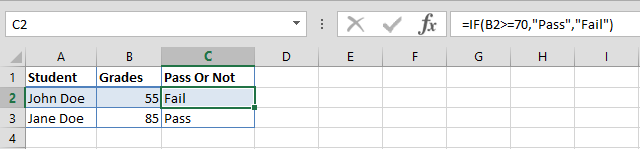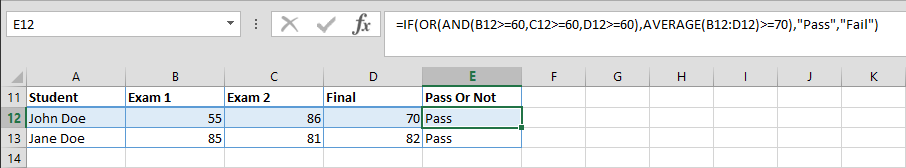The beauty of Excel lies in its flexibility to create pretty much any mathematical model with little effort. Logic gates can be established within a table, chart, or any other spreadsheet element to dissect data and results. This gives Excel users the ability to create advanced data models. In the heart of logic statements lie 4 functions, IF, AND, OR, and NOT. Using these alone, you can automate data. In this article, we’re going to be focusing on their basics, and how to use them. You can download our spreadsheet template below.

# IF

Once of the most popular function in Excel, the IF function, checks a logical statement, and returns a value based on the result. This function has 3 arguments and its parameters are,

=IF(do this logical test, if the answer is true – do this, if answer is false – do this)

Any ‘do this or that’ situation can be given as an example. Here, we created a list of students and their grades. Let’s try to find out who passed and who failed the test. If the grade is equal to or greater than 70 (B2>=70) it’s a pass, otherwise a fail. We can also have Excel print “Pass” if this statement is true and “Fail” if not. The formula then becomes,

=IF(B2>=70,"Pass","Fail")# AND - OR - NOT

IF isn’t the only function that can be used to run logical tests. For when there’s more the than one condition, there are other functions we can utilize. The most common examples are the AND, OR and NOT functions.

These functions, just like the IF function, evaluate a logical statement, and return values based on the outcome. This makes them really handy when using in conjunction with the logical test portion of an IF function. You can think of them as math operators.

• AND returns TRUE only if every argument is true, otherwise gives FALSE.
• OR returns TRUE if any argument is TRUE; if every arguments are FALSE, returns FALSE.
• NOT returns the opposite logical value of the argument. TRUE to FALSE and FALSE to TRUE.

Let’s take a closer look at each function.

## AND

In an alternative scenario, let’s say the students have a total of 3 grades, from 2 exams and a final. A student can pass only if each individual grade is greater than or equal to 60. Here our rule is, "all grades should be greater than or equal to 60”. We can use the AND function to combine these requirements,

=IF(AND(B7>=60,C7>=60,D7>=60),"Pass","Fail")## OR

Let’s take a different case. If either one rule below was enough for a pass grade, we could utilize the OR function.

1. All grades should be equal to or greater than 60 (AND(B7>=60,C7>=60,D7>=60))

OR

1. Average of grades should be equal to or greater than 70 (AVERAGE(B7:D7)>=70)

The statement becomes,

=IF(OR(AND(B7>=60,C7>=60,D7>=60),AVERAGE(B7:D7)>=70),"Pass","Fail")## NOT

When you need to include all results but a certain rule, the NOT function is the best way to go. For example, “All grades should be equal to or greater than 60” statement can be defined as,

=NOT(OR(B17<60,C17<60,D17<60))

This statement will check for any grade that is less than 60. For those that are, the formula returns the opposite parameter (FALSE) because that is the “Fail” condition for this scenario. Looking for a Pass/Fail, the full statement would be as follows,

=IF(OR(NOT(OR(B17<60,C17<60,D17<60)),NOT(AVERAGE(B17:D17)<70)),"Pass","Fail")